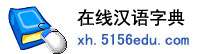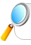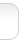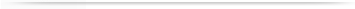汉字   按部首检索  按拼音检索丁肇 邴肇 高肇 赵肇 谢肇

 肇字的男姓名 | 肇字的女姓名 | 肇开头的姓名 | 肇结尾的姓名 | 更多带肇的姓名
 肇基 (男) 肇华 (男) 肇军 (男) 肇东 (男) 肇宏 (男) 肇祥 (男) 肇杰 (男) 肇辉 (男) 肇坤 (男) 肇兴 (男) 肇平 (男) 肇伟 (男) 肇荣 (男) 肇文 (男) 肇林 (男) 肇峰 (男) 肇强 (男) 肇庆 (男) 肇敏 (男) 肇勇 (男) 肇明 (男) 肇英 (女) 肇 (男) 肇霞 (女) 肇宁 (男) 肇民 (男) 肇斌 (男) 肇亮 (男) 肇祺 (男) 肇良 (男) 肇飞 (男) 肇星 (男) 肇禹 (男) 肇雄 (男) 肇全 (男) 肇梁 (男) 肇龙 (男) 肇光 (男) 肇泉 (男) 肇超 (男) 肇敏 (女) 肇 (女) 肇波 (男) 肇康 (男) 肇麟 (男) 肇初 (男) 肇楠 (男) 肇燕 (女) 肇勤 (男) 肇瑞 (男) 肇一 (男) 肇鹏 (男) 肇铎 (男) 肇嘉 (男) 肇武 (男) 肇南 (男) 肇元 (男) 肇和 (男) 肇朗 (男) 新肇 (男) 肇珊 (女) 肇凤 (男) 肇森 (男) 肇海 (男) 肇贤 (男) 肇秋 (女) 肇胜 (男) 秦肇 (男) 肇桢 (男) 肇辉 (女) 肇宁 (女) 肇琼 (女) 肇君 (男) 肇蓉 (女) 肇新 (男) 肇成 (男) 肇年 (男) 肇炀 (男) 肇君 (女) 肇旭 (男) 肇清 (男) 肇均 (男) 肇丽 (女) 肇阳 (男) 肇剑 (男) 肇中 (男) 肇平 (女) 肇玲 (女) 肇毅 (男) 肇杏 (男) 肇洪 (男) 肇炜 (男) 肇伦 (男) 肇锋 (男) 福肇 (男) 肇礼 (男) 燕肇 (男) 肇春 (男) 肇升 (男) 肇忠 (男) 肇先 (男) 挺肇 (男) 肇隆 (男) 肇雯 (女) 忠肇 (男) 肇欣 (男) 肇鑫 (女) 肇箐 (女) 肇玉 (女) 肇显 (男) 肇骏 (男) 肇夏 (女) 肇聚 (男) 肇煜 (男) 肇富 (男) 肇佳 (男) 肇得 (男) 肇欣 (女) 肇学 (男) 肇丰 (男)

 版权所有　在线汉语字典   浙ICP备05019169号